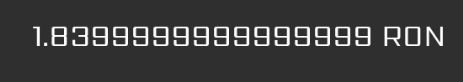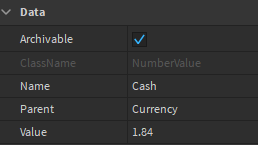# Values showing 1.8399999... when in a GUI, but in the properties it is 1.84

Greetings,

I don’t really know how to explain the problem, but in the GUI the value is shown with a lot of 9s, but in the properties of the value, it is shown correctly. Please help me! (This is happening after I add/take from the NumberValue from a script, such as buying an item)

The GUI:The Value:2 Likes

Ah precision errors strike again, try rounding to the nearest hundredth on your GUI.

Try the solution of this post

BTW Here is the solution to that post fixed to your use case

``````
local roundDecimals = function(num, places) --num is your number or value and places is number of decimal places, in your case you would need 2

places = math.pow(10, places or 0)
num = num * places

if num >= 0 then
num = math.floor(num + 0.5)
else
num = math.ceil(num - 0.5)
end

return num / places

end

local textUi = pathToText

textUi.Text = roundDecimals(pathToNumberValue.Value, 2)

``````
1 Like

You definitely want to round the numbers, if there isn’t a specific reason why this happens, the best way to fix it is by rounding.

Yep, it works very well! Thank you a lot!

1 Like